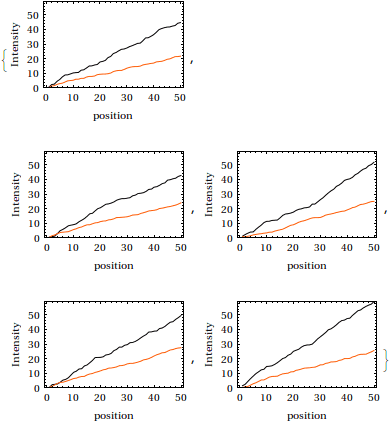# Plotting Nested Lists in Mathematica

• Mathematica
Gold Member
All,

I am having trouble plotting nested lists.

I have two data sets (A and B), both with dimensions 5x50.

So far I have

plot = Map[
ListPlot[#, PlotRange -> {0, Max[A]},
AxesOrigin -> {0, 0}, Frame -> True,
FrameLabel -> {"position", "Intensity"}] &, A];

which gives me 5 plots of 50 points each. What I need is to also plot data B together with data A, ending with 5 plots each having two lines of 50 points.

Each plot is ListPlot[An,Bn] where n=1..5 but I just can't figure it out.

Many thanks for any help and apologies for trespassing upon your time.

Christina

## Answers and Replies

Simon_Tyler
I think that you just need to transpose your data:

Code:
A = Accumulate /@ RandomReal[{0, 2}, {5, 50}];
B = Accumulate /@ RandomReal[{0, 1}, {5, 50}];

ListPlot[#, PlotRange -> {0, Max[A]}, AxesOrigin -> {0, 0},
Frame -> True, Joined -> True,
FrameLabel -> {"position", "Intensity"}] & /@ Transpose[{A, B}]You can also get the same results using Table and Part ( [[ ]] )

Code:
Table[ListPlot[{A[[i]], B[[i]]}, PlotRange -> {0, Max[A]},
AxesOrigin -> {0, 0}, Frame -> True, Joined -> True,
FrameLabel -> {"position", "Intensity"}], {i, 1, 5}]

#### Attachments

Gold Member
It worked perfectly, just what I was looking for.

Many thanks for your help.

Christina